## Biomedical Research

All submissions of the EM system will be redirected to Online Manuscript Submission System. Authors are requested to submit articles directly to Online Manuscript Submission System of respective journal.

Research Article - Biomedical Research (2017) Volume 28, Issue 6

## Hybrid medical image fusion using wavelet and curvelet transform with multi-resolution processing

Sivakumar N1* and Helenprabha K2

1Research Scholar, Anna University, India

2Professor, Department of CSE, RMD Engineering College, India

*Corresponding Author:
Sivakumar N
Research Scholar, Anna University, India

Accepted on November 12, 2016

Visit for more related articles at Biomedical Research

### Abstract

Medical image fusion combines the complementary images from different modalities and enhances the quality of fused output image that provides more anatomical and functional information to the experts. In this paper, a new hybrid approach is proposed to combine the images obtained from Computer Tomography and Magnetic Resonance Imaging using Wavelet and Curvelet Transform Techniques. Alongside, sub-band coding, scale search algorithm was also been used for filtering and image scaling operations was used for decomposition. Ridgelet Transform techniques was used along with radon Transform to perform image transition from 1-D to 2-D during reconstruction stage of fused image. The resulting image was evaluated using objective metrics of Entropy (H), Root Mean Square Error (RMSE) and Peak Signal to Noise Ratio (PSNR). The results showed that the proposed approach has resulted better information in terms of undertaken performance than the existing methods.

## Keywords

Medical image fusion, Hybrid fusion, Wavelet transform, Curvelet transform, Sub-band coding, Scaling algorithm, Ridgelet transform, Radon transform.

## Introduction

Image fusion has increasingly become the promising area of research and has also attracted the researchers over the years, and its importance continually on the rising side . In general, image fusion is a process of combining or merging two or more images of the same scene to enhance the content of the resulting image. Image fusion can be performed at three different levels on a given image or images: signal level or pixel level, feature level and decision level. Numerous image fusion studies has been carried out by the researchers in both spatial and transform domains with different fusion rules such as pixel and weighted average, region variance, maximum value selection and more [2-6]. The main objective of image fusion is to provide resulting image information with increased accuracy and reliability to improve the reading efficiency of both structural and functional information of images .

In mathematical perspective, an image can be seen in two dimensional arrays of intensity values with locally varying statistics which results from different combinations of abrupt features such as edges and contrasting homogeneous regions. The most difficult task with local histograms is making statistical modelling over the span of an entire image as histograms can vary from one part of an image to another .

Various Image fusion techniques were proposed such as PCA, HIS, Averaging technique, and pyramid based, wavelet based, curvelet based, contourlet based transform techniques to serve and support various applications such as medical imaging, satellite imaging, robot vision, battlefield monitoring and remote sensing areas of research and so forth. Each of these techniques has their own merits and demerits when used for different types of image enhancement purposes .

Medical image fusion

Medical image fusion is a process of registering and combining two or more images of same or different imaging modalities to enhance the image quality by reducing randomness and redundancy of the source images . In simple terms, it aims to improve the clinical accuracy of images and its applicability for diagnosis, assessment of medical problems and surgical planning tasks requiring the segmentation, feature extraction, and visualization of multi-modal datasets. The resulting output image should (1) preserve the relevant information from each input image modality, (2) reduce noise and avoid irrelevant information of input image, (3) not to generate distortion and to attain consistency and accuracy in the fused output image .

Hybrid medical image fusion

Medical image fusion is having several imaging modalities which can be used as primary inputs to analyse the undertaken study. But, the most difficult part of the study is the selection of image modality and fusion method for the targeted clinical study. Moreover, having one image modality would not ensure any accuracy, robustness and reliability for the analysis and resulting diagnosis. Therefore, in order to improve the accuracy and robustness of the analysis and resulting diagnosis of the study more image modalities were considered. Furthermore, the researchers were also convinced that looking at images from multiple modalities can offer them better results in terms of reducing randomness, redundancy and improving accuracy, robustness and reliability. As a result, the resulting assessment information is more reliable and accurate [12,13].

In hybrid fusion, the advantages of various different fusion techniques and rules were integrated to obtain single fused output image with better quality results by minimizing Mean Square Value and maximizing signal to noise ratio value .

Proposed approach

This part of the paper discusses about image modalities and methods considered for the undertaken research and workflow of the proposed approach.

Image modalities used

Magnetic Resonance Imaging (MRI) plays vital role in non-invasive diagnosis of brain tumors where segmentation is used to extract different types of tissues and to identify abnormal regions of input images but, it suffers of relative sensitivity to movement which makes it inefficient in assessing organs that involves movement such as mouth tumors. Though, there are various methodological advancements such as Structure Similarity Match Measure (SSIM) in improving the fusion of MR images, this study has considered tissue classification. It is widely known that MR images are highly efficient in presenting soft tissue structures in organs such as brain, heart and eyes with better accuracy [15,16].

Computerized Tomography (CT) technique has a major impact on medical diagnosis and assessments due to its short scan times and high imaging resolutions. Unlike MR images, a CT image reveals more information about the hard tissues as its scan process transverse into every slices of human brain or skull etc . Therefore, the advantages of both MRI and CT images can be combined to bring better results by adopting image addition operation. Arithmetic image fusion is a commonly used scheme which applies a weight (Wn) to each input image (In) and combines these two to form a single output image (f),(1)

In most cases, weights are assigned to give an average effect (i.e.Wn=1/n). This research study has considered MRI and CT scanned images of human brain as input images.

Transform techniques and methods used

Wavelet transform: Wavelet based methods extract the high frequency information from one image in both time and space domains and inject it into another. Wavelet methods decompose images into one low frequency sub band and three high frequency sub bands. These sub bands are combined using inverse wavelet transforms (Figure 1).

It is widely used in signal processing communication applications as it leads to fast transform and represents the information in convenient tree structure. In general, wavelet methods use various mathematical models to perform injection starting from simple addition and aggregate operation to more complex mathematical models. With this approach, the image resolution remains same before and after fusion, but when combined with neural networks and support vector machines, wavelet method offered better results at feature levels of information [18-20].

Curvelet transform: Curvelet transform method is based on segmentation which divides the input image into number of small overlapping tiles and ridegelet transform is applied to each of the tiles to perform edge detection. The resulting output image provides more information by preventing image denoising. Curvelet transform results in better performance than wavelet transform in terms of signal to noise ratio value . The following stages were performed in curvelet transform method (Figure 2).

Sub-band coding: The objective of sub-band coding is to perform Multi-Resolution Analysis (MRA), where input images are decomposed into various band limited components using digital filters, called as sub-bands. 2D sub-band coding was used for decomposing the input image into sub-bands. In general, a system that isolates certain frequency is called a filter, namely low pass filter, high pass filter and band pass filter (Figures 3 and 4).

Low pass filter lets through image components below a certain frequency (f0), high pass filters blocks image components below a certain frequency (f0) and band pass filter lets through the image components lies at the frequencies (f1, f2). Figure 2 represents the sub-band process of 2D filters. Sub-band decomposition process can be defined mathematically as follows,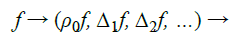(2)

Tiling: This method is used to split the image into overlapping tiles, where the same will then be used for transformation of curved lines that are present in the sub-bands into straight lines, which helps in handling curved edges. A scale selection algorithm  is employed in the proposed approach which modifies the default curvelet choice of the number of scales J. This algorithm ensures that centered high frequency region is smoothed i.e. generally; this part of the inner curvelet scale is not smoothened by the angular divisions. The scale selection’s algorithms optimum scale is given by,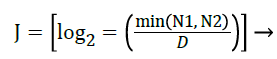(3)

Where, D represents the length of the square covering the high frequency magnitude values,

N1 and N2 represent the number of horizontal and vertical image pixels. The curvelet decomposition levels in default is given by,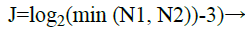(4)

Continuous Ridgelet transform: The ridgelet are an orthogonal set {ρλ} for L2 (radius 2) and it divides the frequency domain to dyadic coronae |ξ| ϵ [2s, 2s+1]. It samples the coronae in two ways: in radical direction, where wavelets are used for sampling; in angular direction, samples the sth coronae at least 2 s times (Figure 5).

Using this method of transform, image coefficients can be represented as f (x1, x2), function can be mathematically written as follows: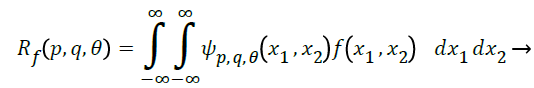(5)

Where,Ѱ is a basic ridgelet function where a>0 and ϵ [0, 2π] is constant .

The biggest drawback of ridgelet transform is that it works only for line singularities. To overcome this issue, projection slice theorem of radon transform is combined with ridgelet transform to transform line singularities to point singularities. So, radon transform for an image, f is the collection of integrals indexed by is defined as,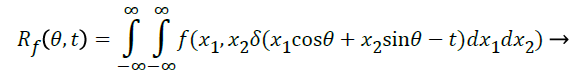(6)

When combining the equation (5) with (6), we get the following: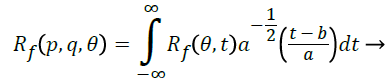(7)

## Result Analysis and Evaluation

Basically, Image fusion method evaluation can be done in two ways, namely, subjective and objective. In general, Image fusion metrics such as entropy, mutual information, peak signal to noise ratio, root mean square error, structural similarity index, and universal image quality index were considered for the objective analysis of implemented image fusion method.

Subjective analysis is of more concerned in showing the undertaken input image and fused output image to the field of experts in medicine. The experts will analyse the images and give feedback in terms of accuracy and reliability with respect to anatomical and functional information seen in the fused image output .

Performance metrics

There are various number of objective metrics exists of varying degree of complexity and a host of different approaches. Our research work has considered the following three metrics to evaluate the performance of the proposed approach.

Entropy (H): The entropy value used to determine the information content of the fused image in comparison with the input image i.e. higher value of H represents better information and lower value of H represents the minimum information. Entropy was calculated using the following equation,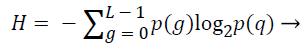(8)

Root mean square error (RMSE): This metric measures the difference between the input reference image with the fused output image and is calculated with the following equation,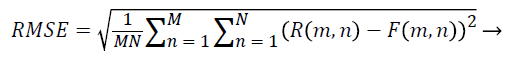(9)

Where M, N represents the dimensions of images, R(m, n) represents the reference of input images and F(m, n) represents the references of fused output image.

Peak signal to noise ratio (PSNR): It represents the ratio between the maximum power of a signal and the percentage of corrupting noise that affects the reliability of its representation. It can be mathematically measured as follows,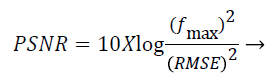(10)

## Result Analysis

As mentioned earlier in this paper, hybrid image fusion has been proposed and the same has been implemented using two input images, CT and MRI respectively. The information obtained by the fused resultant image was verified with three metrics, namely, entropy, MSE and PSNR. Both the input images used for image fusion and the resultant fused image are presented below (Figures 6a-6g). The performance metrics and its value information are presented on Table 1. From Table 1, it is clear that the proposed algorithm performs better than other methods.

Performance Metrics Transform Techniques
Wavelet Curvelet Agarwal Hybrid
Entropy 9.31 8.94 8.81 8.76
Root mean square error 3.83 3.53 3.31 3.29
Peak signal to noise ratio 32.73 38.09 41.91 43.1

Table 1: Metric information values for various fusion methods.

## Conclusion

Though, there have been too many image fusion methods available to enhance the features of a resulting fused image, there still exist an engineering need to develop more efficient hybrid methods that could result in improved accuracy and reliability. From the obtained results, it is evident that the proposed approach has delivered better results in comparison with wavelet, curvelet methods alone. In addition, the results were better when compared with the hybrid fusion method proposed by Agarwal in . In our future work, a different scaling algorithm will be considered along with other possible fusion methods such as contourlet transform, wave atom transform etc.